千年恋：寻兽危途

正文第六章——黑衣人出现

[更新时间] 2019-07-13 06:42:53 [字数] 1393

云依喃喃自语道：“胡杨树呢，怎么会没有了？”云依想不明白满眼失望。-=-+^本书=首&发纵%横!中文网^^=\$-

“姑娘，你现在可以告诉我你在找什么了吗？也许我可以帮你。”-=-+^本书=首&发纵%横!中文网^^=\$-

“我在找我昨天的梦。”-=-+^本书=首&发纵%横!中文网^^=\$-

“昨天的梦，什么梦还可以找回来？”对于云依的回答，魏队的反应并不惊讶反倒有些高兴。-=-+^本书=首&发纵%横!中文网^^=\$-

云依眼里含着泪，看着魏队说道：“说出来也许你不信，我昨天在前面不远的地方真的看到一棵胡杨树，可今天怎么就没有了呢？好大的一棵胡杨树被火烧的黑漆漆的，还有一个和我长得一模一样的女孩，被捆在那颗树上，树都烧光了女孩却没被烧到，不过她还是死了，她死后天上下起黄沙雨，漫天的黄沙把那个女孩埋在沙子下面，还有很多人都被埋在沙子下面，不要用海市蜃楼来诓我，我还从那颗胡杨树的树杆上取下一个镯子。”-=-+^本书=首&发纵%横!中文网^^=\$-

“你看！”云依伸出手让魏队看自己戴在手上的镯子。-=-+^本书=首&发纵%横!中文网^^=\$-

“哦，对了我还看到一个和你长得也很像的人，那个人好像是个将军，被一道光带走了，没有被埋在沙子下面。”-=-+^本书=首&发纵%横!中文网^^=\$-

“姑娘，还不知道你叫什么名字。”-=-+^本书=首&发纵%横!中文网^^=\$-

“云依。”云依的回答又让魏队心头一紧。-=-+^本书=首&发纵%横!中文网^^=\$-

“姑娘，你昨天一定没到雅丹其它地方看过吧？我带你欣赏一下雅丹的美景如何？”-=-+^本书=首&发纵%横!中文网^^=\$-

“好呀。”至少可以弥补损失，昨天只看一个景点就莫名其妙的睡到晚上，门票和租车钱都算白花了，今天有人愿意再带她重游雅丹，她怎么可以拒绝。-=-+^本书=首&发纵%横!中文网^^=\$-

“姑娘，你梦里那棵胡杨树，千年前确实存在过，不过不在雅丹，它在孔雀河岸，你手上的镯子也不是普通的镯子，它是神物它找到你说明你是它的主人，只有主人出现镯子才会出现。” -=-+^本书=首&发纵%横!中文网^^=\$-

“什么？”云依听了魏队的话有点懵！感觉像天方夜谭，-=-+^本书=首&发纵%横!中文网^^=\$-

“那……”云依还没想好说什么，就被魏队打断话接过去：“姑娘如果对镯子的故事有兴趣，我带姑娘去三危山，给姑娘讲讲镯子的故事好吗？”-=-+^本书=首&发纵%横!中文网^^=\$-

云依，本不是一个爱与人亲近的人，这次陌生人的邀请她竟然同意了？-=-+^本书=首&发纵%横!中文网^^=\$-

这是她潜意识的反应，她的潜意识告诉她魏队是可以信赖的，潜意识还告诉她，跟着魏队可以解答她心里的疑惑。-=-+^本书=首&发纵%横!中文网^^=\$-

地下一个黑衣男子高举双手紧握双拳激动地说：“镯子的主人出现了，你终于出现了，我被困在这里已有千年了！”-=-+^本书=首&发纵%横!中文网^^=\$-

“啪”一声黑衣人一拳砸在石凳上，凳子裂成两半。-=-+^本书=首&发纵%横!中文网^^=\$-

-=-+^本书=首&发纵%横!中文网^^=\$-

-=-+^本书=首&发纵%横!中文网^^=\$-

-=-+^本书=首&发纵%横!中文网^^=\$-

-=-+^本书=首&发纵%横!中文网^^=\$-

-=-+^本书=首&发纵%横!中文网^^=\$-

(快捷键：←)上一章 回目录(快捷键：回车) 下一章(快捷键：→)

发表评论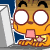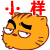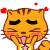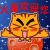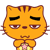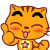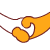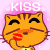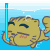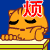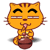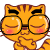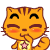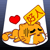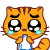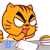12